# GMAT Math : Calculating the slope of a perpendicular line

## Example Questions

### Example Question #1 : Calculating The Slope Of A Perpendicular Line

What is the slope of the line perpendicular to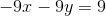?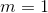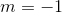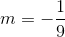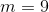Explanation:

Perpendicular lines have slopes that are negative reciprocals of each other. Therefore, rewrite the equation in slope intercept form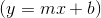: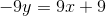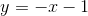Slope of given line:Negative reciprocal:### Example Question #2 : Calculating The Slope Of A Perpendicular Line

Line 1 is the line of the equation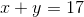. Line 2 is perpendicular to this line. What is the slope of Line 2?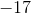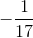Explanation:

Rewrite in slope-intercept form: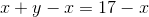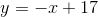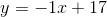The slope of the line is the coefficient of, which is. A line perpendicular to this has as its slope the opposite of the reciprocal of: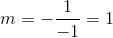### Example Question #2 : Calculating The Slope Of A Perpendicular Line

Given: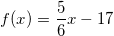Calculate the slope of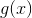, a line perpendicular to.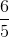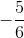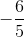Explanation:

To find the slope of a line perpendicular to a given line, simply take the opposite reciprocal of the slope of the given line.

Since f(x) is given in slope intercept form,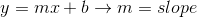.

Therefore our original slope is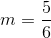So our new slope becomes: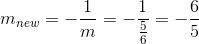### Example Question #4 : Calculating The Slope Of A Perpendicular Line

What would be the slope of a line perpendicular to the following line?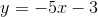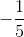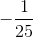Explanation:

The equation for a line in standard form is written as follows:Whereis the slope of the line andis the y intercept. By definition, the slope of a line is the negative reciprocal of the slope of the line to which it is perpendicular. So if the given line has a slope of, the slope of any line perpendicular to it will have the negative reciprocal of that slope. This gives us: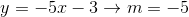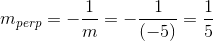### Example Question #1 : Calculating The Slope Of A Perpendicular Line

What is the slope of a line perpendicular to the line of the equation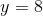?The line has an undefined slope.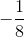The line has an undefined slope.

Explanation:

The graph of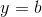for any real numberis a horizontal line. A line parallel to it is a vertical line, which has a slope that is undefined.

### Example Question #6 : Calculating The Slope Of A Perpendicular Line

Give the slope of a line on the coordinate plane.

Statement 1: The line shares an-intercept and its-intercept with the line of the equation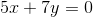.

Statement 2: The line is perpendicular to the line of the equation.

Statement 1 ALONE is sufficient to answer the question, but Statement 2 ALONE is NOT sufficient to answer the question.

BOTH statements TOGETHER are sufficient to answer the question, but NEITHER statement ALONE is sufficient to answer the question.

BOTH statements TOGETHER are insufficient to answer the question.

EITHER statement ALONE is sufficient to answer the question.

Statement 2 ALONE is sufficient to answer the question, but Statement 1 ALONE is NOT sufficient to answer the question.

Statement 2 ALONE is sufficient to answer the question, but Statement 1 ALONE is NOT sufficient to answer the question.

Explanation:

Assume Statement 1 alone. In order to determine the slope of a line on the coordinate plane, the coordinates of two of its points are needed. The-intercept of the line of the equation can be found by substituting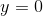and solving for: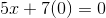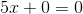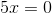The-intercept of the line is at the origin,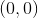. It follows that the-intercept is also at the origin. Therefore, Statement 1 only gives one point on the line, and its slope cannot be determined.

Assume Statement 2 alone. The slope of the line of the equationcan be calculated by putting it in slope-intercept form: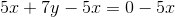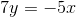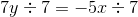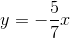The slope of this line is the coefficient of, which is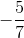. A line perpendicular to this one has as its slope the opposite of the reciprocal of, which is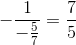.